# Andhra Pradesh SSC Board Question Paper for Class 10th Maths Paper 2 2015 In PDF

## AP SSC or 10th Class Question Paper Mathematics Paper 2 English Medium 2015 with Solutions – Free Download

Andhra Pradesh SSC (Class 10) Maths 2015 question paper 2 with solutions are given here in a downloadable pdf format and also in the text so that the students can easily get them. Along with the solutions, they can also access the Maths question paper 2 2015 Class 10 SSC for reference. Students are able to access all the Andhra Pradesh board previous year maths question papers here. AP 10th Class Mathematics Question Paper 2015 Paper 2 can be downloaded easily, and students can practice and verify the answers provided by BYJU’S. Solving 2015 Maths question paper 2 for Class 10 will help the students to predict the type of questions that will appear in the exam.

### QUESTION PAPER CODE 16E(A)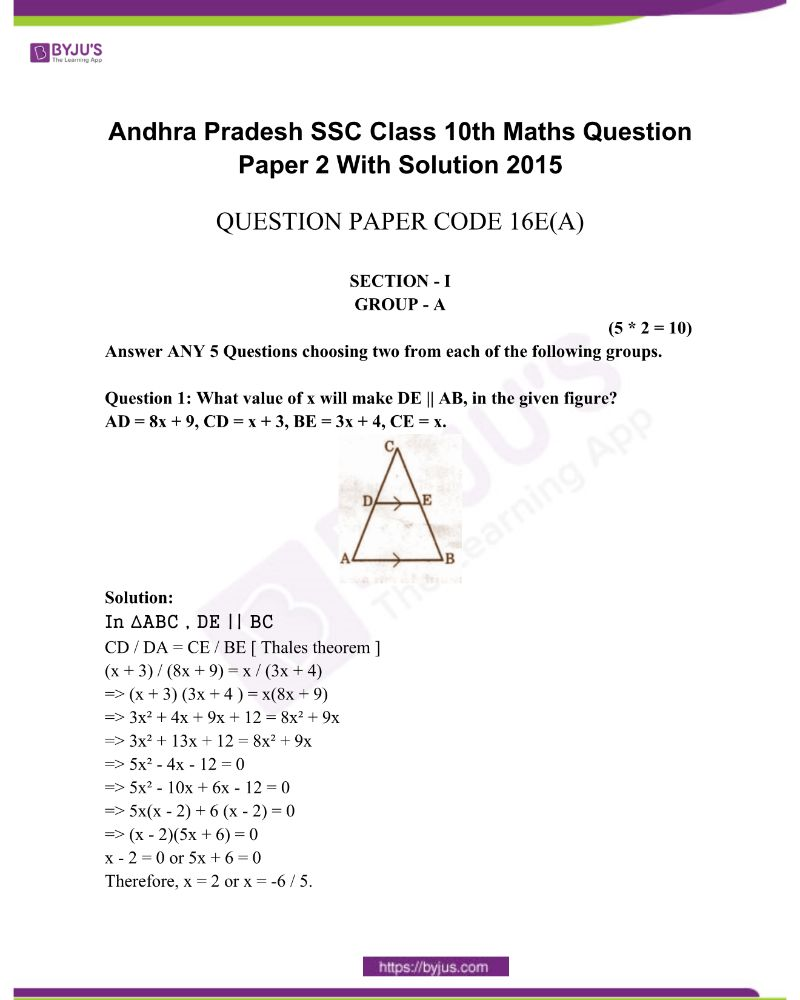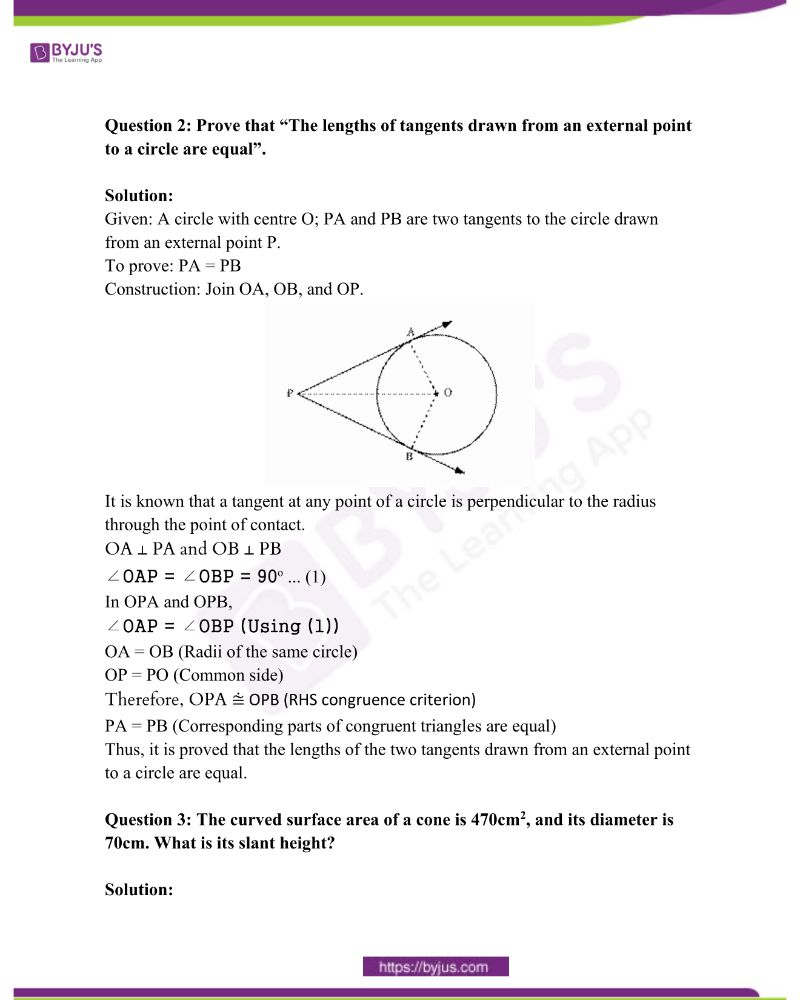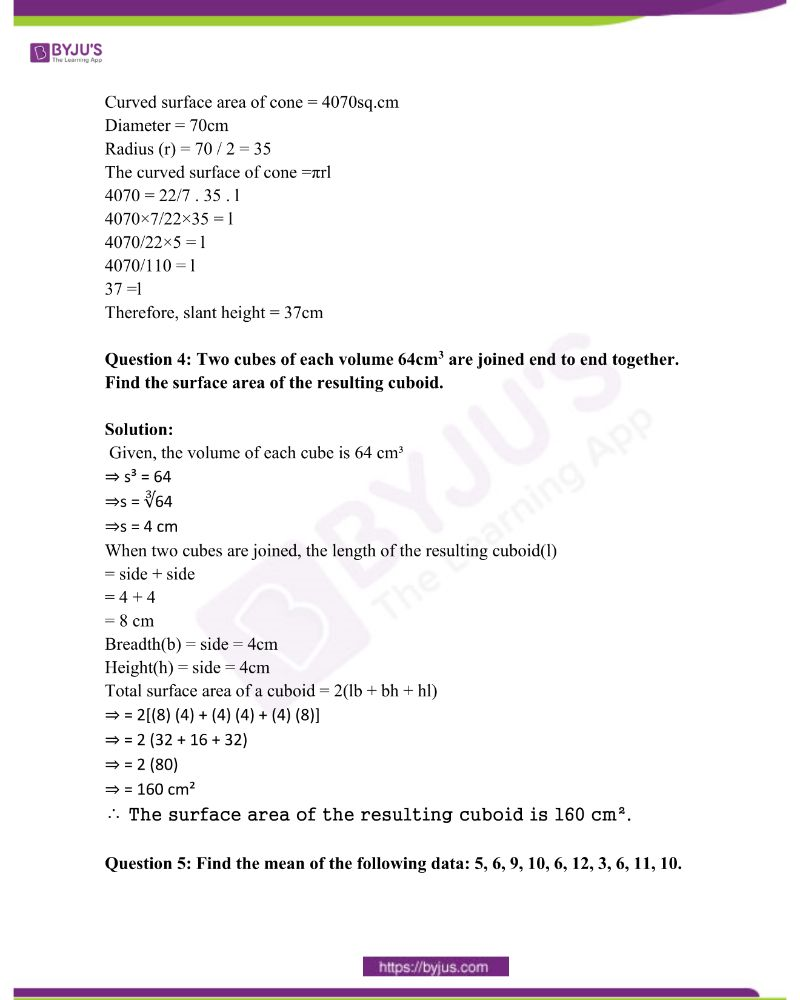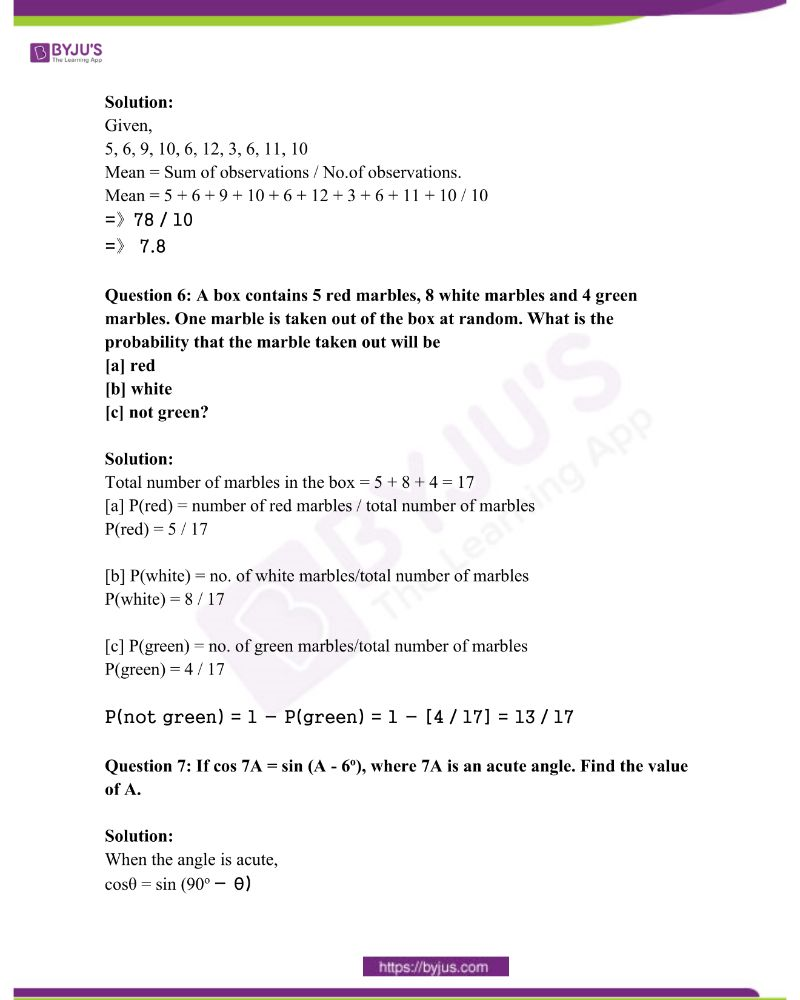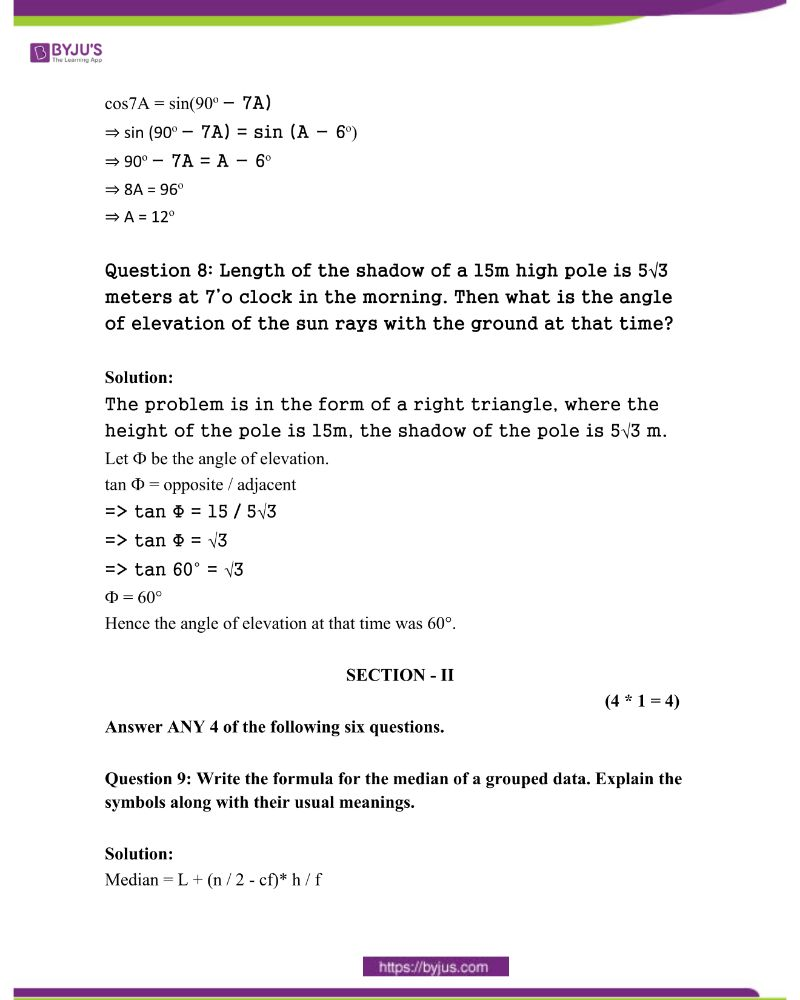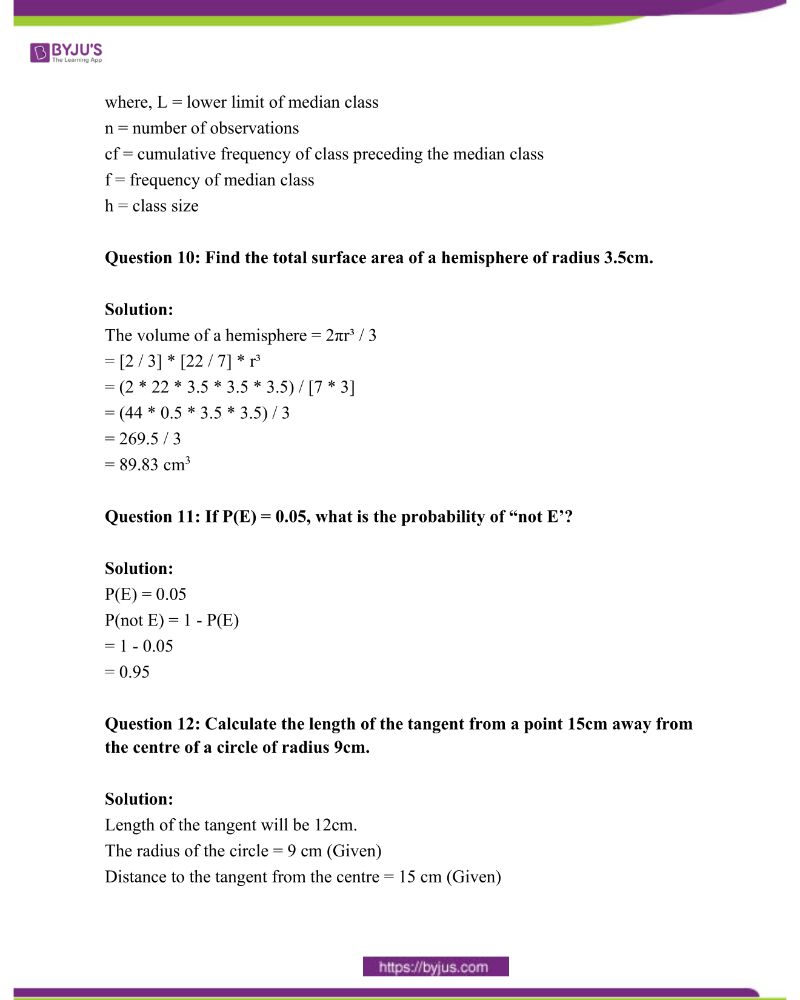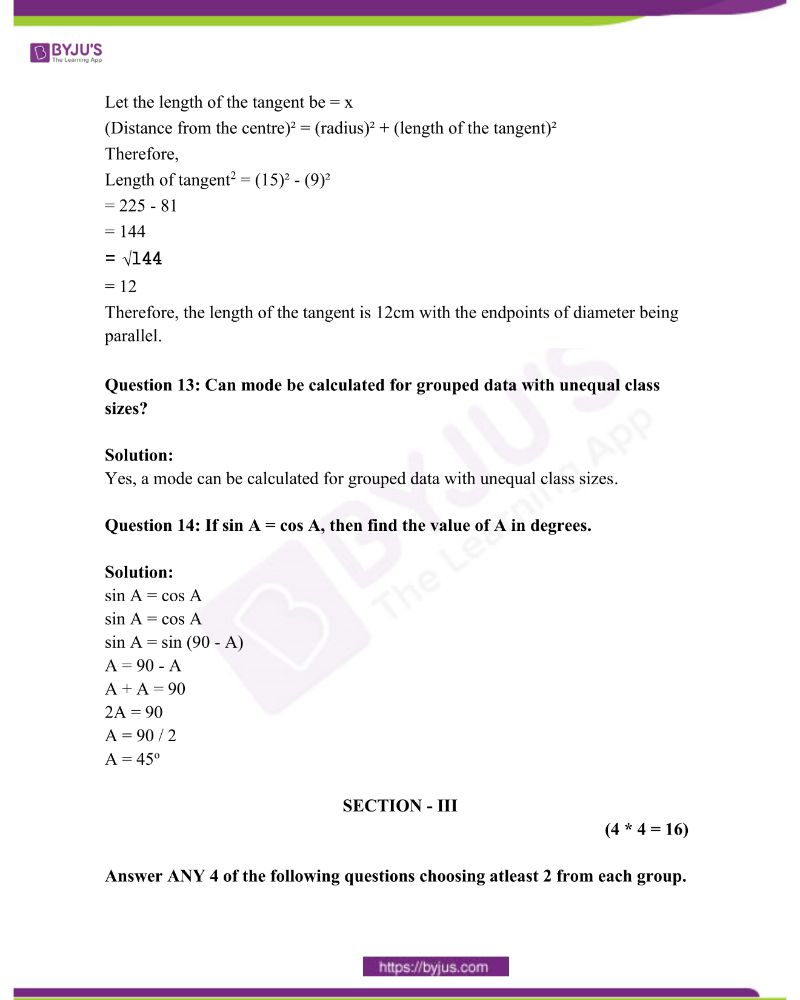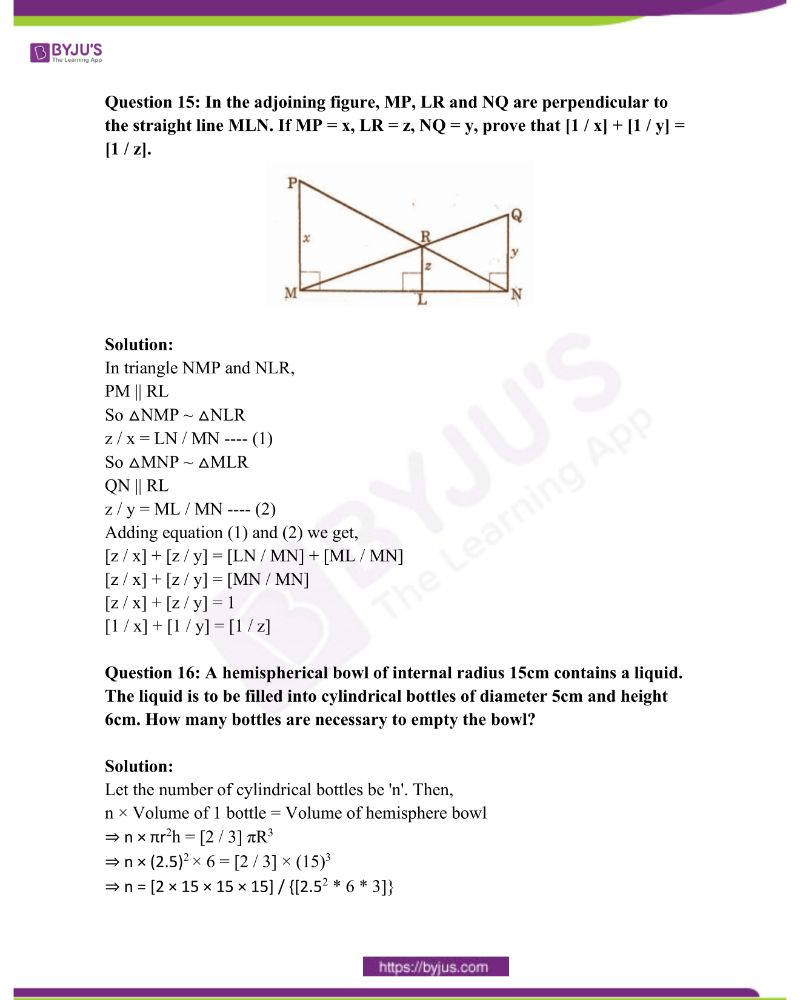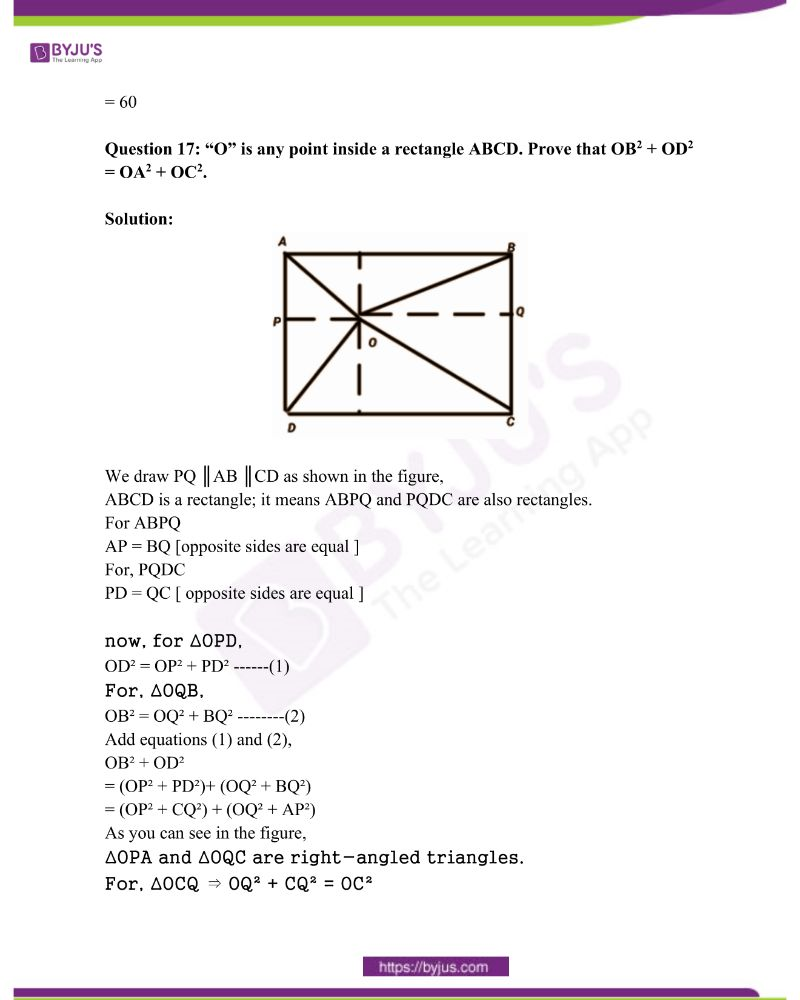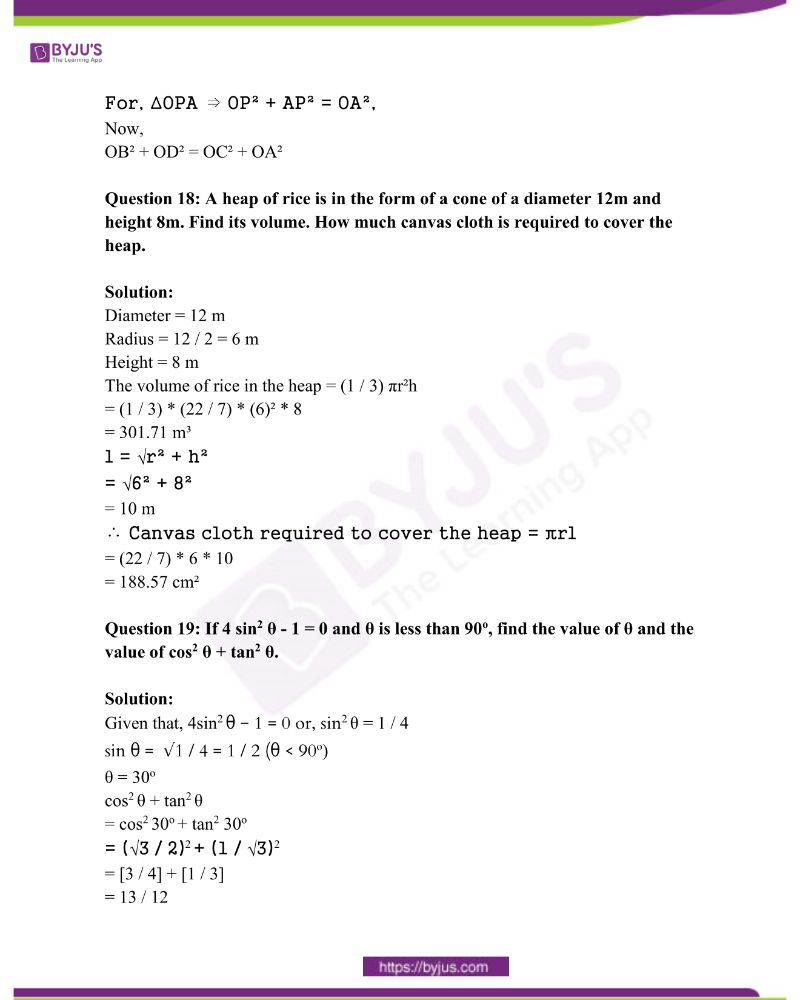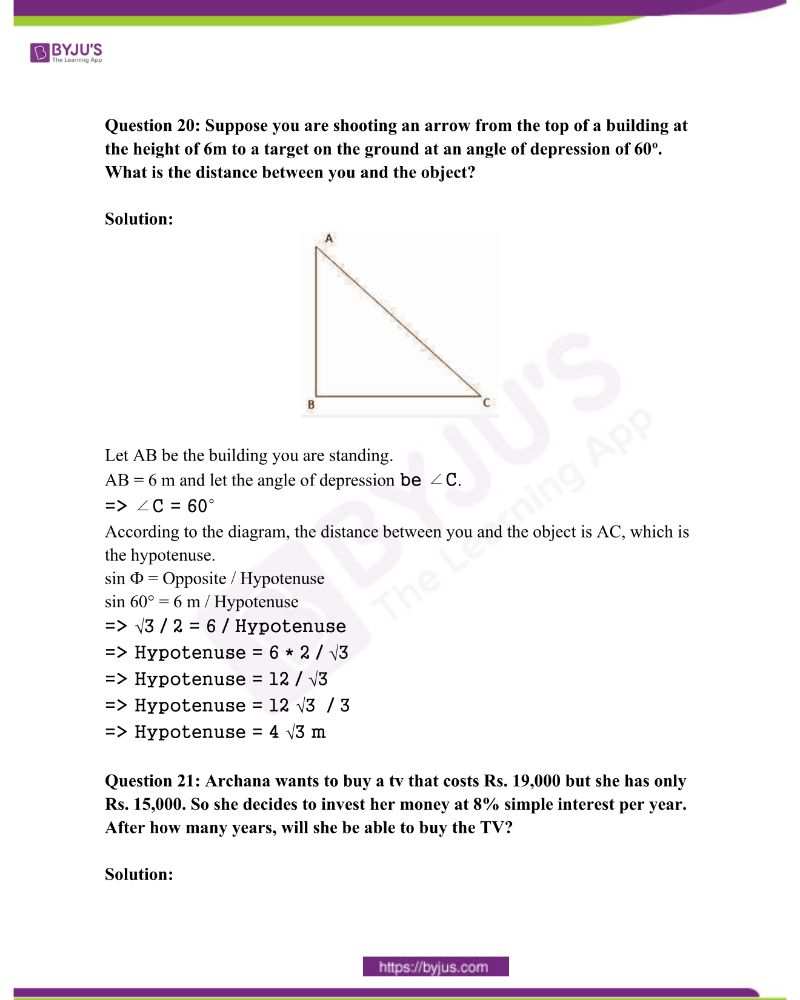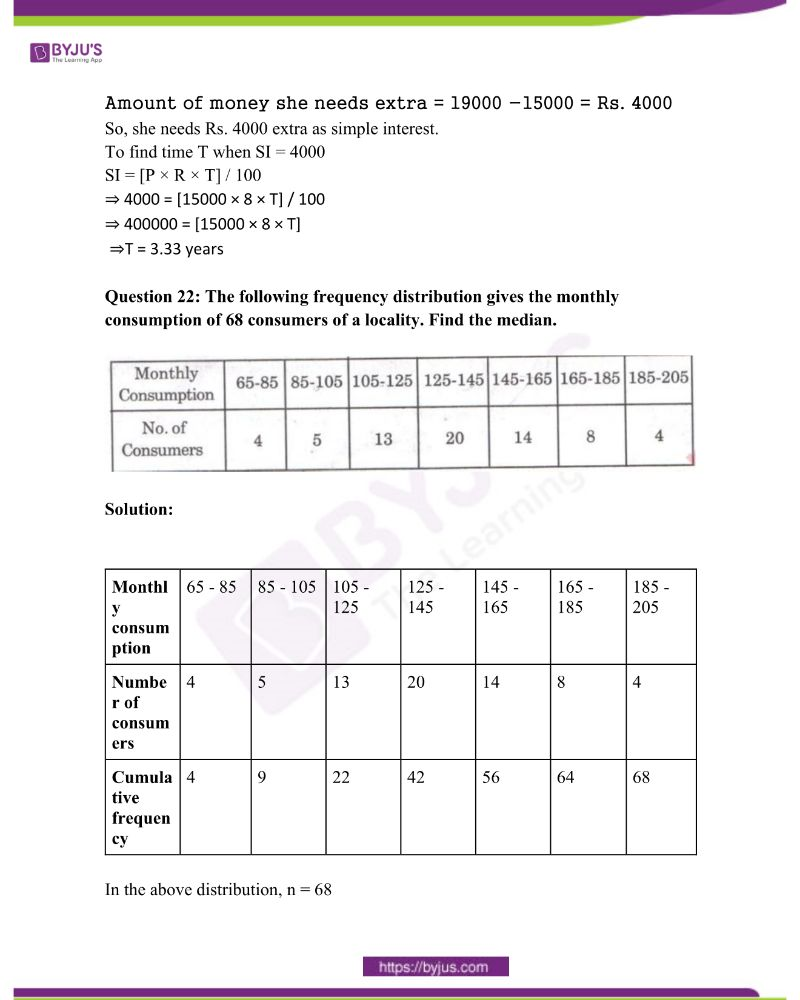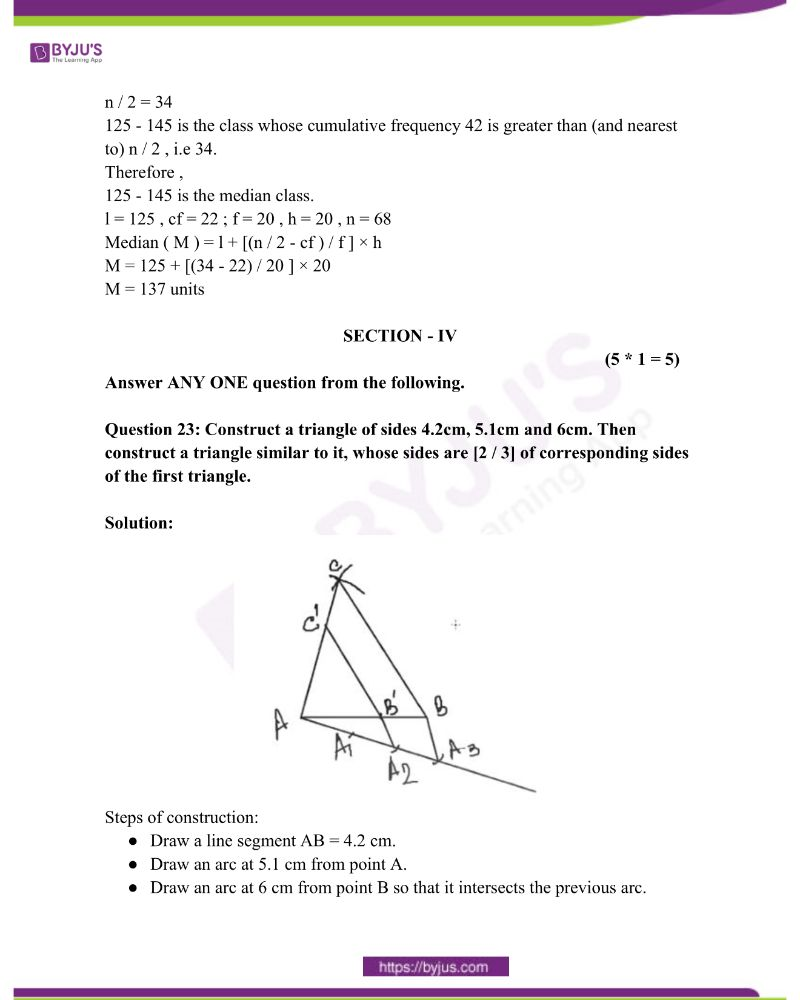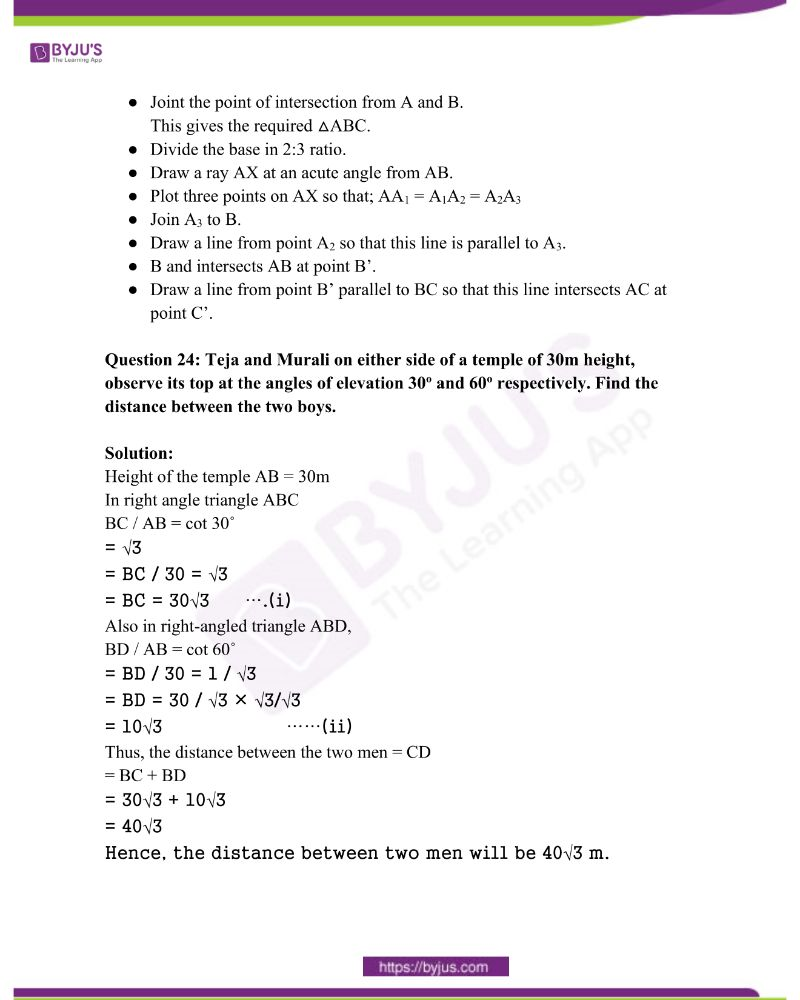SECTION – I

GROUP – A

(5 * 2 = 10)

Answer ANY 5 Questions choosing two from each of the following groups.

Question 1: What value of x will make DE || AB, in the given figure?

AD = 8x + 9, CD = x + 3, BE = 3x + 4, CE = x.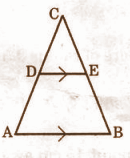Solution:

In ∆ABC , DE || BC

CD / DA = CE / BE [ Thales theorem ]

(x + 3) / (8x + 9) = x / (3x + 4)

=> (x + 3) (3x + 4 ) = x(8x + 9)

=> 3x² + 4x + 9x + 12 = 8x² + 9x

=> 3x² + 13x + 12 = 8x² + 9x

=> 5x² – 4x – 12 = 0

=> 5x² – 10x + 6x – 12 = 0

=> 5x(x – 2) + 6 (x – 2) = 0

=> (x – 2)(5x + 6) = 0

x – 2 = 0 or 5x + 6 = 0

Therefore, x = 2 or x = -6 / 5.

Question 2: Prove that “The lengths of tangents drawn from an external point to a circle are equal”.

Solution:

Given: A circle with centre O; PA and PB are two tangents to the circle drawn from an external point P.

To prove: PA = PB

Construction: Join OA, OB, and OP.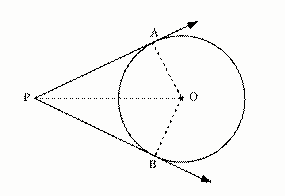It is known that a tangent at any point of a circle is perpendicular to the radius through the point of contact.

OA ⟂ PA and OB ⟂ PB

∠OAP = ∠OBP = 90o … (1)

In OPA and OPB,

∠OAP = ∠OBP (Using (1))

OA = OB (Radii of the same circle)

OP = PO (Common side)

Therefore, OPA ⩭ OPB (RHS congruence criterion)

PA = PB (Corresponding parts of congruent triangles are equal)

Thus, it is proved that the lengths of the two tangents drawn from an external point to a circle are equal.

Question 3: The curved surface area of a cone is 470cm2, and its diameter is 70cm. What is its slant height?

Solution:

Curved surface area of cone = 4070sq.cm

Diameter = 70cm

Radius (r) = 70 / 2 = 35

The curved surface of cone =πrl

4070 = 22/7 . 35 . l

4070×7/22×35 = l

4070/22×5 = l

4070/110 = l

37 =l

Therefore, slant height = 37cm

Question 4: Two cubes of each volume 64cm3 are joined end to end together. Find the surface area of the resulting cuboid.

Solution:

Given, the volume of each cube is 64 cm³

⇒ s³ = 64

⇒s = ∛64

⇒s = 4 cm

When two cubes are joined, the length of the resulting cuboid(l)

= side + side

= 4 + 4

= 8 cm

Height(h) = side = 4cm

Total surface area of a cuboid = 2(lb + bh + hl)

⇒ = 2[(8) (4) + (4) (4) + (4) (8)]

⇒ = 2 (32 + 16 + 32)

⇒ = 2 (80)

⇒ = 160 cm²

∴ The surface area of the resulting cuboid is 160 cm².

Question 5: Find the mean of the following data: 5, 6, 9, 10, 6, 12, 3, 6, 11, 10.

Solution:

Given,

5, 6, 9, 10, 6, 12, 3, 6, 11, 10

Mean = Sum of observations / No.of observations.

Mean = 5 + 6 + 9 + 10 + 6 + 12 + 3 + 6 + 11 + 10 / 10

=》78 / 10

=》 7.8

Question 6: A box contains 5 red marbles, 8 white marbles and 4 green marbles. One marble is taken out of the box at random. What is the probability that the marble taken out will be

[a] red

[b] white

[c] not green?

Solution:

Total number of marbles in the box = 5 + 8 + 4 = 17

[a] P(red) = number of red marbles / total number of marbles

P(red) = 5 / 17

[b] P(white) = no. of white marbles/total number of marbles

P(white) = 8 / 17

[c] P(green) = no. of green marbles/total number of marbles

P(green) = 4 / 17

P(not green) = 1 − P(green) = 1 – [4 / 17] = 13 / 17

Question 7: If cos 7A = sin (A – 6o), where 7A is an acute angle. Find the value of A.

Solution:

When the angle is acute,

cosθ = sin (90o − θ)

cos7A = sin(90o − 7A)

⇒ sin (90o − 7A) = sin (A − 6o)

⇒ 90o − 7A = A − 6o

⇒ 8A = 96o

⇒ A = 12o

Question 8: Length of the shadow of a 15m high pole is 5√3 meters at 7’o clock in the morning. Then what is the angle of elevation of the sun rays with the ground at that time?

Solution:

The problem is in the form of a right triangle, where the height of the pole is 15m, the shadow of the pole is 5√3 m.

Let Ф be the angle of elevation.

tan Ф = opposite / adjacent

=> tan Ф = 15 / 5√3

=> tan Ф = √3

=> tan 60° = √3

Ф = 60°

Hence the angle of elevation at that time was 60°.

SECTION – II

(4 * 1 = 4)

Answer ANY 4 of the following six questions.

Question 9: Write the formula for the median of a grouped data. Explain the symbols along with their usual meanings.

Solution:

Median = L + (n / 2 – cf)* h / f

where, L = lower limit of median class

n = number of observations

cf = cumulative frequency of class preceding the median class

f = frequency of median class

h = class size

Question 10: Find the total surface area of a hemisphere of radius 3.5cm.

Solution:

The volume of a hemisphere = 2πr³ / 3

= [2 / 3] * [22 / 7] * r³

= (2 * 22 * 3.5 * 3.5 * 3.5) / [7 * 3]

= (44 * 0.5 * 3.5 * 3.5) / 3

= 269.5 / 3

= 89.83 cm3

Question 11: If P(E) = 0.05, what is the probability of “not E’?

Solution:

P(E) = 0.05

P(not E) = 1 – P(E)

= 1 – 0.05

= 0.95

Question 12: Calculate the length of the tangent from a point 15cm away from the centre of a circle of radius 9cm.

Solution:

Length of the tangent will be 12cm.

The radius of the circle = 9 cm (Given)

Distance to the tangent from the centre = 15 cm (Given)

Let the length of the tangent be = x

(Distance from the centre)² = (radius)² + (length of the tangent)²

Therefore,

Length of tangent2 = (15)² – (9)²

= 225 – 81

= 144

= √144

= 12

Therefore, the length of the tangent is 12cm with the endpoints of diameter being parallel.

Question 13: Can mode be calculated for grouped data with unequal class sizes?

Solution:

Yes, a mode can be calculated for grouped data with unequal class sizes.

Question 14: If sin A = cos A, then find the value of A in degrees.

Solution:

sin A = cos A

sin A = cos A

sin A = sin (90 – A)

A = 90 – A

A + A = 90

2A = 90

A = 90 / 2

A = 45o

SECTION – III

(4 * 4 = 16)

Answer ANY 4 of the following questions choosing atleast 2 from each group.

Question 15: In the adjoining figure, MP, LR and NQ are perpendicular to the straight line MLN. If MP = x, LR = z, NQ = y, prove that [1 / x] + [1 / y] = [1 / z].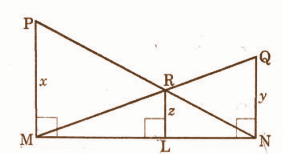Solution:

In triangle NMP and NLR,

PM || RL

So △NMP ~ △NLR

z / x = LN / MN —- (1)

So △MNP ~ △MLR

QN || RL

z / y = ML / MN —- (2)

Adding equation (1) and (2) we get,

[z / x] + [z / y] = [LN / MN] + [ML / MN] [z / x] + [z / y] = [MN / MN] [z / x] + [z / y] = 1

[1 / x] + [1 / y] = [1 / z]

Question 16: A hemispherical bowl of internal radius 15cm contains a liquid. The liquid is to be filled into cylindrical bottles of diameter 5cm and height 6cm. How many bottles are necessary to empty the bowl?

Solution:

Let the number of cylindrical bottles be ‘n’. Then,

n × Volume of 1 bottle = Volume of hemisphere bowl

⇒ n × πr2h = [2 / 3] πR3

⇒ n × (2.5)2 × 6 = [2 / 3] × (15)3

⇒ n = [2 × 15 × 15 × 15] / {[2.52 * 6 * 3]}

​= 60

Question 17: “O” is any point inside a rectangle ABCD. Prove that OB2 + OD2 = OA2 + OC2.

Solution: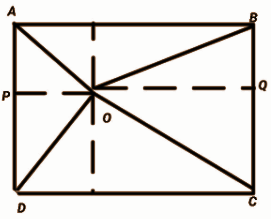We draw PQ ║AB ║CD as shown in the figure,

ABCD is a rectangle; it means ABPQ and PQDC are also rectangles.

For ABPQ

AP = BQ [opposite sides are equal ]

For, PQDC

PD = QC [ opposite sides are equal ]

now, for ∆OPD,

OD² = OP² + PD² ——(1)

For, ∆OQB,

OB² = OQ² + BQ² ——–(2)

OB² + OD²

= (OP² + PD²)+ (OQ² + BQ²)

= (OP² + CQ²) + (OQ² + AP²)

As you can see in the figure,

∆OPA and ∆OQC are right-angled triangles.

For, ∆OCQ ⇒ OQ² + CQ² = OC²

For, ∆OPA ⇒ OP² + AP² = OA²,

Now,

OB² + OD² = OC² + OA²

Question 18: A heap of rice is in the form of a cone of a diameter 12m and height 8m. Find its volume. How much canvas cloth is required to cover the heap.

Solution:

Diameter = 12 m

Radius = 12 / 2 = 6 m

Height = 8 m

The volume of rice in the heap = (1 / 3) πr²h

= (1 / 3) * (22 / 7) * (6)² * 8

= 301.71 m³

l = √r² + h²

= √6² + 8²

= 10 m

∴ Canvas cloth required to cover the heap = πrl

= (22 / 7) * 6 * 10

= 188.57 cm²

Question 19: If 4 sin2 θ – 1 = 0 and θ is less than 90o, find the value of θ and the value of cos2 θ + tan2 θ.

Solution:

Given that, 4sin2 θ − 1 = 0 or, sin2 θ = 1 / 4

sin θ = √1 / 4 = 1 / 2 (θ < 90o)

θ = 30o

cos2 θ + tan2 θ

= cos2 30o + tan2 30o

= (√3 / 2)2 + (1 / √3)2

= [3 / 4] + [1 / 3]

= 13 / 12

Question 20: Suppose you are shooting an arrow from the top of a building at the height of 6m to a target on the ground at an angle of depression of 60o. What is the distance between you and the object?

Solution: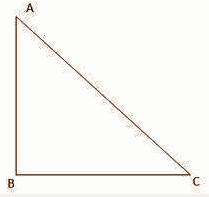Let AB be the building you are standing.

AB = 6 m and let the angle of depression be ∠C.

=> ∠C = 60°

According to the diagram, the distance between you and the object is AC, which is the hypotenuse.

sin Ф = Opposite / Hypotenuse

sin 60° = 6 m / Hypotenuse

=> √3 / 2 = 6 / Hypotenuse

=> Hypotenuse = 6 * 2 / √3

=> Hypotenuse = 12 / √3

=> Hypotenuse = 12 √3 / 3

=> Hypotenuse = 4 √3 m

Question 21: Archana wants to buy a tv that costs Rs. 19,000 but she has only Rs. 15,000. So she decides to invest her money at 8% simple interest per year. After how many years, will she be able to buy the TV?

Solution:

Amount of money she needs extra = 19000 −15000 = Rs. 4000

So, she needs Rs. 4000 extra as simple interest.

To find time T when SI = 4000

SI = [P × R × T] / 100

⇒ 4000 = [15000 × 8 × T] / 100

⇒ 400000 = [15000 × 8 × T]

⇒T = 3.33 years

Question 22: The following frequency distribution gives the monthly consumption of 68 consumers of a locality. Find the median.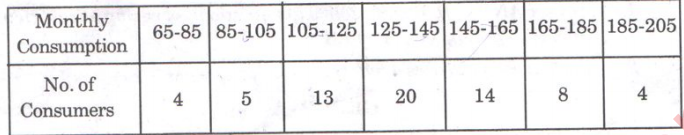Solution:

 Monthly consumption 65 – 85 85 – 105 105 – 125 125 – 145 145 – 165 165 – 185 185 – 205 Number of consumers 4 5 13 20 14 8 4 Cumulative frequency 4 9 22 42 56 64 68

In the above distribution, n = 68

n / 2 = 34

125 – 145 is the class whose cumulative frequency 42 is greater than (and nearest to) n / 2 , i.e 34.

Therefore ,

125 – 145 is the median class.

l = 125 , cf = 22 ; f = 20 , h = 20 , n = 68

Median ( M ) = l + [(n / 2 – cf ) / f ] × h

M = 125 + [(34 – 22) / 20 ] × 20

M = 137 units

SECTION – IV

(5 * 1 = 5)

Answer ANY ONE question from the following.

Question 23: Construct a triangle of sides 4.2cm, 5.1cm and 6cm. Then construct a triangle similar to it, whose sides are [2 / 3] of corresponding sides of the first triangle.

Solution: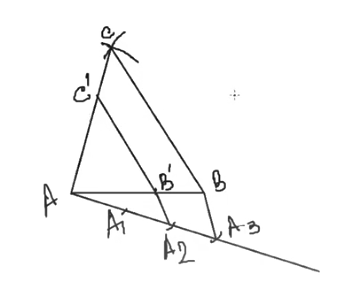Steps of construction:

• Draw a line segment AB = 4.2 cm.
• Draw an arc at 5.1 cm from point A.
• Draw an arc at 6 cm from point B so that it intersects the previous arc.
• Joint the point of intersection from A and B.

This gives the required △ABC.

• Divide the base in 2:3 ratio.
• Draw a ray AX at an acute angle from AB.
• Plot three points on AX so that; AA1 = A1A2 = A2A3
• ​Join A3 to B.
• Draw a line from point A2 so that this line is parallel to A3.
• B and intersects AB at point B’.
• Draw a line from point B’ parallel to BC so that this line intersects AC at point C’.

Question 24: Teja and Murali on either side of a temple of 30m height, observe its top at the angles of elevation 30o and 60o respectively. Find the distance between the two boys.

Solution:

Height of the temple AB = 30m

In right angle triangle ABC

BC / AB = cot 30˚

= √3

= BC / 30 = √3

= BC = 30√3 ….(i)

Also in right-angled triangle ABD,

BD / AB = cot 60˚

= BD / 30 = 1 / √3

= BD = 30 / √3 × √3/√3

= 10√3 ……(ii)

Thus, the distance between the two men = CD

= BC + BD

= 30√3 + 10√3

= 40√3

Hence, the distance between two men will be 40√3 m.